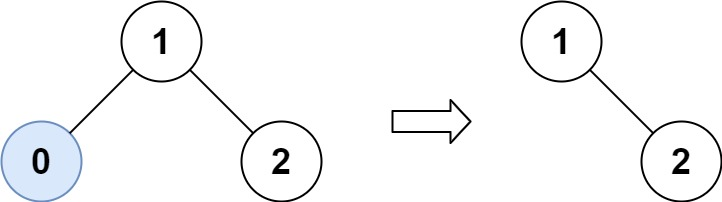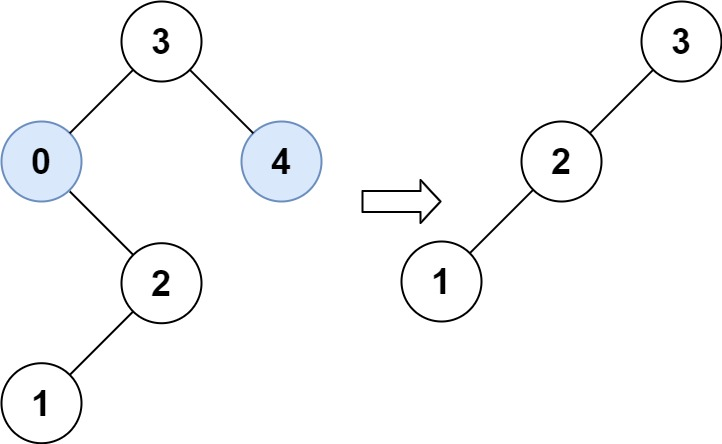# 669. Trim a Binary Search Tree

Medium
Given the `root` of a binary search tree and the lowest and highest boundaries as `low` and `high`, trim the tree so that all its elements lies in `[low, high]`. Trimming the tree should not change the relative structure of the elements that will remain in the tree (i.e., any node's descendant should remain a descendant). It can be proven that there is a unique answer.
Return the root of the trimmed binary search tree. Note that the root may change depending on the given bounds.
Example 1:Input: root = [1,0,2], low = 1, high = 2
Output: [1,null,2]
Example 2:Input: root = [3,0,4,null,2,null,null,1], low = 1, high = 3
Output: [3,2,null,1]
Constraints:
• The number of nodes in the tree is in the range `[1, 10^4]`.
• `0 <= Node.val <= 10^4`
• The value of each node in the tree is unique.
• `root` is guaranteed to be a valid binary search tree.
• `0 <= low <= high <= 10^4`

### 解題

Runtime: 6 ms, faster than 100%
Memory Usage: 6.4 MB, less than 100%
/**
* Definition for a binary tree node.
* type TreeNode struct {
* Val int
* Left *TreeNode
* Right *TreeNode
* }
*/
func trimBST(root *TreeNode, low int, high int) *TreeNode {
if root == nil { return nil }
if root.Val < low {
return trimBST(root.Right, low, high)
} else if root.Val > high {
return trimBST(root.Left, low, high)
}
return &TreeNode{ Val: root.Val, Left: trimBST(root.Left, low, high), Right: trimBST(root.Right, low, high) }
}